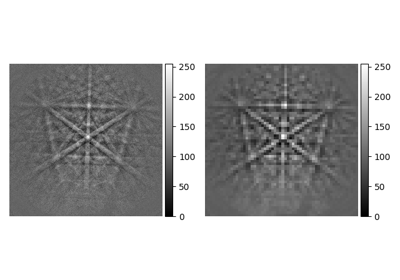# downsample#

EBSD.downsample(factor: int, dtype_out: = None, show_progressbar: = None, inplace: bool = True, lazy_output: = None) [source]#

Downsample the pattern shape by an integer factor and rescale intensities to fill the data type range.

Parameters:
factor

Integer binning factor to downsample by. Must be a divisor of the initial pattern shape $$(s_y, s_x)$$. The new pattern shape given by the factor $$k$$ is $$(s_y / k, s_x / k)$$.

dtype_out

Name of the data type of the new patterns overwriting data. Contrast between patterns is lost. If not given, patterns maintain their data type and. Patterns are rescaled to fill the data type range.

show_progressbar

Whether to show a progressbar. If not given, the value of hyperspy.api.preferences.General.show_progressbar is used.

inplace

Whether to operate on the current signal or return a new one. Default is True.

lazy_output

Whether the returned signal is lazy. If not given this follows from the current signal. Can only be True if inplace=False.

Returns:
s_out

Downsampled signal, returned if inplace=False. Whether it is lazy is determined from lazy_output.

Notes

This method differs from rebin() in that intensities are rescaled after binning in order to maintain the data type. If rescaling is undesirable, use rebin() instead.

## Examples using EBSD.downsample#Pattern binning

Pattern binning